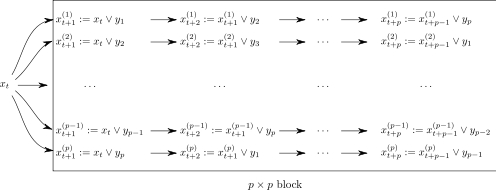Want to share your content on R-bloggers? click here if you have a blog, or here if you don't.Given the growing interest in parallel processing through GPUs or multiple processors, there is a clear need for a proper use of (uniform) random number generators in this environment. We were discussing the issue yesterday with Jean-Michel Marin and briefly looked at a few solutions:

• given p parallel streams/threads/processors, starting each generator with a random seed provides no guarantee for independence between the sequences;
• since most random generators are periodic with humongous periods T, starting the i-th sequence at$s_{iT/p}$ should see no overlap between the sequences if p is not enormous. But finding the (iT/p)-th value of the sequence is not necessarily feasible;
• using a single starting seed$s_0$, allocating each new value$s_t$ to the i-th processor if$t=i text{mod}(p)$ is ensuring independence between the sequences, but it sounds contradictory with parallel computing requirements. However, if the p-step version$Psi^p$ of the generator can be derived [and coded], we simply need to start the i-th sequence at$s_i$ and use transforms of this starting point by$Psi^p$. This is actually a well-known method that goes under the name of the leapfrog technique, proposed by Bowman and Robinson in 1987 at a SIAM conference (taking place in Philadelphia, of all places!). There are subsequent papers in the literature that cast doubt on the performances of this technique, but I do not see why… Since the original pseudo-random generator is assumed to be correct, (a) taking a subsequence from the main sequence should preserve the uniformity and independence, and (b) the subsequence should have a long enough period if p remains within hundreds..

Obviously, we did not do any serious search in the recent literature and there are many other approaches to be found in the computer literature, including the scalable parallel random number generation (SPRNG) packages of Michael Mascagni (including an R version) and Matsumoto’s dynamic creator, a C program that provides starting values for independent streams when using the Mersenne twister.